# Test: Ratio And Proportion, Indices, Logarithms - 2

## 40 Questions MCQ Test Quantitative Aptitude for CA CPT | Test: Ratio And Proportion, Indices, Logarithms - 2

Description
Attempt Test: Ratio And Proportion, Indices, Logarithms - 2 | 40 questions in 40 minutes | Mock test for CA Foundation preparation | Free important questions MCQ to study Quantitative Aptitude for CA CPT for CA Foundation Exam | Download free PDF with solutions
QUESTION: 1

Solution:
QUESTION: 2

Solution:
QUESTION: 3

### The numbers 14, 16, 35, 42 are not in proportion. The fourth term for which they will be in proportion is

Solution:
QUESTION: 4

If x/y = z/w, implies y/x = w/z, then the process is called

Solution:
QUESTION: 5

If p/q = r/s = p–r/q–s, the process is called

Solution:
QUESTION: 6

If a/b = c/d, implies (a+b)/(a–b) = (c+d)/(c–d), the process is called

Solution:
QUESTION: 7

If u/v = w/p, then (u–v)/(u+v) = (w–p)/(w+p). The process is called

Solution:
QUESTION: 8

12, 16, *, 20 are in proportion. Then * is

Solution:
QUESTION: 9

4, *, 9, 13½ are in proportion. Then * is

Solution:
QUESTION: 10

The mean proportional between 1.4 gms and 5.6 gms is

Solution:

Let 1.4 gms, x gms and 5.6 gms be in continuous proportion.

(Mean proportional terms)^2 = Product of extremes.

Therefore x^2 = 1.4 x 5.6

therefore x^2 = 7.84

therefore x= 2.8 gms.

QUESTION: 11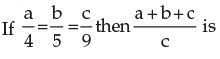Solution:
QUESTION: 12

Two numbers are in the ratio 3 : 4; if 6 be added to each terms of the ratio, then the new ratio will be 4 : 5, then the numbers are

Solution:
QUESTION: 13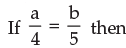Solution:
QUESTION: 14

Two numbers are respectively 20% and 50% more than a third number. The ratio of the two numbers is:

Solution: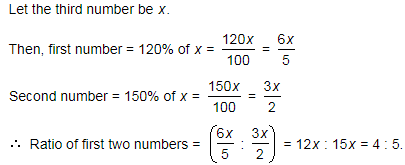QUESTION: 15

if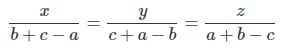then  (b-c)x + (c-a)y+(a-b)z is

Solution:

Let X=(b+c-a);y=(c+a-b);z=(a+b-c)

=(b-c)(b+c-a)+(c-a)(c+a-b)+(a-b)(a+b-c)

=b^2-c^2-ab+ac+c^2-a^2-bc+ab+a^2-b^2-ac+bc

=0

QUESTION: 16

4x–1/4 is expressed as

Solution:
QUESTION: 17

The value of 81/3 is

Solution:
QUESTION: 18

The value of 2 × (32) 1/5 is

Solution:
QUESTION: 19

The value of 4/(32)1/5 is

Solution:
QUESTION: 20

The value of (8/27)1/3 is

Solution:
QUESTION: 21

2½ .4¾ is equal to

Solution:
QUESTION: 22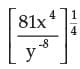has simplified value equal to

Solution:
QUESTION: 23

xa–b × xb–c × xc–a is equal to

Solution:
QUESTION: 24

The value of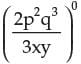is equal to

Solution:
QUESTION: 25

{(33)2 × (42)3 × (53)2} / {(32)3 × (43)2 × (52)3} is

Solution:
QUESTION: 26

Which is True ?

Solution:
QUESTION: 27

If x1/p = y1/q = z1/r and xyz = 1, then the value of p+q+r is

Solution:
QUESTION: 28

The value of ya–b × yb–c × yc–a × y–a–b is

Solution:
QUESTION: 29

The True option is

Solution:
QUESTION: 30

The simplified value of 16x–3y2 × 8–1x3y–2 is

Solution:
QUESTION: 31

The value of (8/27)–1/3 × (32/243)–1/5 is

Solution:
QUESTION: 32

The value of {(x+y)2/3 (x–y)3/2/√x+y × √ (x–y)3}6 is

Solution:
QUESTION: 33

Simplified value of (125)2/3 × √25 × 3√53 × 51/2 is

Solution:
QUESTION: 34

[{(2)1/2 . (4)3/4 . (8)5/6 . (16)7/8 . (32)9/10}4]3/25 is

Solution:
QUESTION: 35

[1–{1–(1–x2)–1}–1]–1/2 is equal to

Solution:

Solution :- [1–{1–(1–x2)–1}–1]–1/2

=[1 - { 1- x2 -1/(1-x2) }-1]-1/2 here we used a-n = 1/an

= [1 - {(-x^2)/(1-x^2)}^-1]^-1/2

= [ 1 - { x^2 /( x^2 -1) }-1]-1/2

= [ 1- (x2-1)/ x2]-1/2

= [ (x2 -x2+1)/ x2]-1/2

= [1/x2]-1/2

= [x2]1/2

= x 2*1/2

= x

QUESTION: 36

Ajay and Raj together have Rs. 1050. On taking Rs. 150 from Ajay, Ajay will have same amount as what Raj had earlier. Find the ratio of amounts with Ajay and Raj initially.

Solution: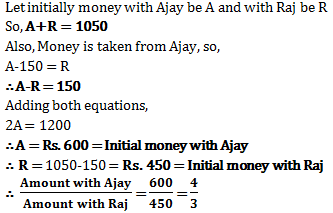QUESTION: 37

If a3–b3 = (a–b) (a2 + ab + b2), then the simplified form of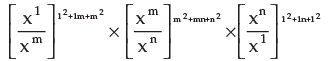Solution:
QUESTION: 38

Using (a–b)3 = a3–b3–3ab(a–b) tick the correct of these when x = p1/3 – p–1/3

Solution:
QUESTION: 39

On simplification, 1/(1+am–n+am–p) + 1/(1+an–m+an–p) + 1/(1+ap–m+ap–n) is equal to

Solution:
QUESTION: 40

If A:B = 2:3, B:C = 4:5 and C:D = 6:7, then find the value of A:B:C:D

Solution: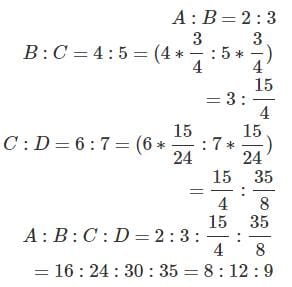Use Code STAYHOME200 and get INR 200 additional OFF Use Coupon Code